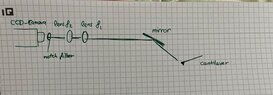# Total focal length and magnification

• I
Aymangh994
I would like to realize an image of the cantilever. The dimensions are (450x50) microns. I would like to use a self-constructed telephoto lens. This consists of two lenses. The image magnification can be 4 to 8. The magnification of the image is nothing more than the ratio between the two focal lengths of the two lenses. E.g. for f1= 320mm f2=40mm the total f= 35.5mm and the imaging magnification is 8 . Since typical focal lenghts of telephoto are over 50mm, I would like to ask if the total focal length matters? I.e. for f1= 800mm and f2=100mm the total focal length is f=88.89 mm but the image magnification remains the same.

•davenn

Gold Member
You need to specify more about the set-up. What is "the cantilever"? We need an annotated diagram if you want sensible answers.
Edit: PS I think you are assuming the 'telescope formula' applies but your object distance is not infinite and are you looking at an image or projecting it onto a sensor?

•davenn and hutchphd
Aymangh994
I would like to ask if the total focal length matters using two different combinations of lenses but the magnification still the same.
Combination 1 : f1= 320mm f2=40mm the total f= 35.5mm and M= 8
Combination 2 : f1= 800mm and f2=100mm the total focal length is f=88.89 mm and M= 8
and how to calculate the working distance ?

Aymangh994
Sketch
following questions :
1. should I use the lensmaker's equation to calculate the working distance ? which focal length should I insert in the equation? the total f or f1 ?
2. The distance between the two lenses and the distance between the CCD-Sensor and the last lens ?

#### Attachments

•WhatsApp Image 2022-09-24 at 00.34.23.jpeg
36.4 KB · Views: 19
•